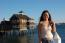Could you please let me know which answer is correct and why? Thanks! A project manager made 3-point estimates on a critical path and found the following results:Assuming ±3 sigma precision level for each estimate, what is the standard deviation of the allover path? App. 4.2 days App. 5.2 days App. 6.2 days You can not derive the path standard deviation from the information given.

### App 5.2 days

1.

To find SD for entire project, add activity variance for all the individual activities and do square root of variance.

2.

To find SD for a series of items, remember the rule, you cannot add SDs; you must first convert the SD into variances, add the variances and then take the SQUARE ROOT of the total and convert back into SD.

In this case,

SD = (Tp-To) / 6                                  V = SD2

 Activity Standard Deviation Variance Activity A 2 4 Activity B 1 1 Activity C 2 4 Activity D 3 9 Activity B 3 9

Vproject = 4 + 1 + 4 + 9 + 9 = 27

SDproject = sqrt(Vproject) =  sqrt(27) = 5.19 ~ 5.2

### Standard Deviation for one

Standard Deviation for one activity = (PE-OE)/6

If you do it for every activity you will get (24-12)/6=2 for actvity A

and similarly 1,2,3,3 for B,C,D,E respectively

Now SD of entire path = square root of total variance

Now total variance =Sum of squares of individual Standard Deviations

So cumilative variance =4+1+4+9+9=27

Now when you take square root of 27 it comes out to be 5.1961 so the answer is approx 5.2 days

### Precision Level

Would the solution have changed if the precision level for each estimate was different?

### Precision

Thanks confused as this made me dig into this word.

http://en.wikipedia.org/wiki/Accuracy_and_precision

I think in estimating context, Accuracy may be the right terminology, ie how much we tend to be nearer to the mean duration/cost value. Precision is more about repeatability of getting the same results, ie measurement uncertainities, To reduce the precision variance, we need  to use more samples. I saw a sample question related to this.

For the exam point of view, I think it is better to understand confidence level, confidence interval, precision and accuracy correctly.

The above question not fully correct in a sense, it mixes PERT which is a beta distrution with 3 sigma which is primaily about normal distrubtion. The argument used is the central limit theorem what considers all the indivdual variances to follow a normal distrubution when they are many data points. So the above calculation needs to have many tasks , infact I I read above 30 or so.. For the exam point of view, its fine if asked to do this way.

Renji

### And with 6 Sigma?

What the answer will be if the estimate is with 6 Sigma ?

And how to calculate it ?

thanks

### calculation for 6 sigma

calculation for 6 sigma

total durationa estimate given = 83.1

for 6 sigma = +/- 6 from total estimate duration

range => (83.1- 6 ) ; (83.1+6)

= 75.1 - 89.1

### I have a question, the

I have a question, the question ask :
"the standard deviation of the allover path"

Standard deviation is (P-O)/6 Why we dont do the sum of all P and O and calculate the Standard Deviation ???

### calculation 6 sigma

this calc is not right,  +/- 6 sigma

a std deviation using 6sig precision would have a slightly lower std deviation of the path.

### The working of standard

The working of standard deviation is perfect one for good working as it needs for good use of things in order. The perfection of things in order leads for perfection of things as it works in order. The source of best assignment is here for quality return in life.